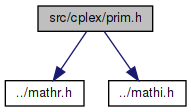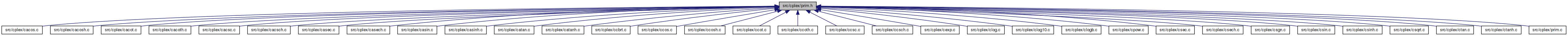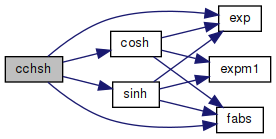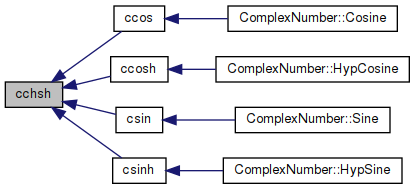amath  1.8.5 Simple command line calculator
prim.h File Reference

Primitives in math library for handling complex numbers. More...

`#include "../mathr.h"`
`#include "../mathi.h"`
Include dependency graph for prim.h:This graph shows which files directly or indirectly include this file:Go to the source code of this file.

## Functions

void cchsh (double x, double *c, double *s)
Calculate cosh and sinh. More...

## Detailed Description

Primitives in math library for handling complex numbers.

Definition in file prim.h.

## ◆ cchsh()

 void cchsh ( double x, double * c, double * s )

Calculate cosh and sinh.

Definition at line 197 of file prim.c.

References cosh(), exp(), fabs(), and sinh().

Referenced by ccos(), ccosh(), csin(), and csinh().

198 {
199  double e, ei;
200
201  if (fabs(x) <= 0.5)
202  {
203  *c = cosh(x);
204  *s = sinh(x);
205  }
206  else
207  {
208  e = exp(x);
209  ei = 0.5 / e;
210  e = 0.5 * e;
211  *s = e - ei;
212  *c = e + ei;
213  }
214 }
double cosh(double x)
Hyperbolic cosine function.
Definition: cosh.c:83
double sinh(double x)
Hyperbolic sine function.
Definition: sinh.c:77
double exp(double x)
Returns the exponential of x.
Definition: exp.c:138
double fabs(double x)
Returns the absolute value of x.
Definition: fabs.c:51
Here is the call graph for this function:Here is the caller graph for this function: